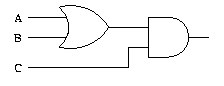# Introduction To IT - Boolean Logic

14 Questions | Total Attempts: 214SettingsCreate your own Quiz• 1.
Simplification of the Boolean expression AB + ABC + ABCD + ABCDE + ABCDEF yields which of the following results?
• A.

ABCDEF

• B.

AB

• C.

A + B + C + D + E + F

• D.

A + B(C+D(E+F))

• 2.
An equivalent representation for the Boolean expression A' + 1 is
• A.

1

• B.

A

• C.

A'

• D.

0

• 3.
Which of the following is the best definition of a literal?
• A.

1 or 2

• B.

The complement of a Boolean variable

• C.

The actual understanding of a Boolean variable

• D.

A Boolean variable

• 4.
Draw a logic table for the diagram above and determine the output.
• A.

No answer is needed. You can skip this question.

• B.

No answer is needed. You can skip this question.

• 5.
The OR function is Boolean multiplication and the AND function is Boolean addition.
• A.

True

• B.

False

• 6.
In Boolean algebra, A + 1 = 1.
• A.

True

• B.

False

• 7.
SOP stands for sum-of-powers
• A.

True

• B.

False

• 8.
The symbol shown below is for a 2-input NAND gate.
• A.

True

• B.

False

• 9.
By applying De Morgan's theorem to a NOR gate, two identical truth tables can be produced.
• A.

True

• B.

False

• 10.
In boolean logic, what symbol can be used to represent AND?
• 11.
Also OR can be represented by?
• 12.
This image represents a                                gate.
• 13.
Which of the following is true of a NOR gate?
• A.

It always yields a positive result.

• B.

It always yields a negative result.

• C.

It is the negative of the OR gate

• D.

It is the same as the OR gate

• 14.
When output is determined solely by output, it is called a                                           logic.
Related TopicsBack to top
×

Wait!
Here's an interesting quiz for you.